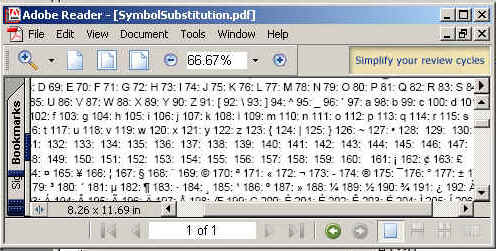Tutorials

# Symbol Substitution

In this example , we are going to explain how we can make symbols using iText api,s. We are using integer values which will converted it into symbol. For this we just convert the integer value into its character value.

In this example , we are going to explain how we can make symbols using iText api,s. We are using integer values which will converted it into symbol. For this we just convert the integer value into its character value.

# Symbol Substitution

In this example , we are going to explain how we can make symbols using iText api,s. We are using integer values which will  converted it into symbol. For this we just convert the integer value into its character value.

Code Description:

In this example we  convents the integer values from 33 to 255 and 913 to 969 into character by using explicit casting  of integer values to character values. We use add(Phrase.getInstance(String s)) method to add the values into the document.

The code of the program is given below:

 ``` import java.io.FileOutputStream; import java.io.IOException; import com.lowagie.text.Document; import com.lowagie.text.DocumentException; import com.lowagie.text.Phrase; import com.lowagie.text.pdf.PdfWriter; import com.lowagie.text.Paragraph; public class SymbolSubstitution {   public static void main(String[] args) throws Exception  {   System.out.println("Symbol Substitution");   Document document = new Document();   PdfWriter.getInstance(document,new  FileOutputStream("SymbolSubstitution.pdf"));   document.open();   document.add(new Paragraph("The values and its  symboles are given below:"));   for (int i = 33; i < 255; i++) {   document.add(Phrase.getInstance(" " + i + ": " + (char) i));  }    for (int i = 913; i < 969; i++) {   document.add(Phrase.getInstance(" " + i + ": " + (char) i));   }   document.close();   } }```

The output of the program is given below: